### Other Maths Pages at this site:

Triangles and Geometry
Pythagorean triangles
Right-angled triangles with integer sides, e.g. 3, 4, 5.
Exact Trig Values for Simple Angles
Which angles have a simple exact value for their sine,cosine or tangent?
A Triangle Convertor for Cartesian, Trilinear and Barycentric Coordinates
introduction to trilinear and barycentric coordinates, links to Clark Kimberling's Encyclopedia of Triangle Centers (ETC) with automatic lookup
Integers
Primes & Factors Calculator
Integer Bases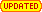many kinds of bases to represent integeres
Integer Palindromes
Polygonal and Figurate Numbers
Triangular, Square, Pentagonal ... Numbers
More on Polygonal Numbers
Central polygonal numbers, matchstick figures, 3D solid shapes and higher dimensions
Runsums
Numbers which are the sum of a run of consecutive whole numbers
More on Runsums
Integer Sums or Partitions of an integer
The number 2016
The number 2021
Fractions
Fractions and Decimals
- their periods and patterns and in non-decimal bases.
Farey Fractions and Stern-Brocot Tree Calculators
Two ways of arranging all fractions
Egyptian fractions
The Egyptians only had unit fractions of the form 1/n. How did they use them?
Introduction to Continued Fractions
An unusual method of writing fractions that has many advantages.
An Exact Fractions Calculator and converter
Fractions to and from Decimal, Continued Fractions from and to Fractions with an expression evaluator and many in-built functions and all to as many decimal places as you like!.
Linear Recurrence Relations and Generating Functions
Games
Lock and Roll!
A Yahtzee-type dice game
Got it!
A Countdown-type numbers game,

## Fibonacci Numbers and the Golden Section

This is the Home page for Dr Ron Knott's multimedia web site on the Fibonacci numbers, the Golden section and the Golden string hosted by the Mathematics Department of the University of Surrey, UK.

The Fibonacci numbers are
0, 1, 1, 2, 3, 5, 8, 13, ... (add the last two to get the next)

The golden section numbers are

0·61803 39887... = phi = ϕ and
1·61803 39887... = Phi = Φ = 1 + phi = 1/phi

The golden string is

1 0 1 1 0 1 0 1 1 0 1 1 0 1 0 1 1 0 1 ...
a sequence of 0s and 1s that is closely related to the Fibonacci numbers and the golden section.

If you want a quick introduction then have a look at the first link on the Fibonacci numbers and where they appear in Nature.

 What's New? - the FIBLOG 15 February 2022 To mark the Chinese New Year a new section on The Remainders Number System has been added to the Number Bases page, based on the Chinese Remainder Theorem. 2 February 2022 The whole of my Maths website at University of Surrey is now secure and accessible by https. You should notice no difference in accessing it. The former page URL: http://www.maths.surrey.ac.uk/hosted-sites/R.Knott/Fibonacci/fib.html will now automatically direct you to this new one. This page is now https://r-knott.surrey.ac.uk/Fibonacci/fib.html All of my other maths pages are under r-knott.surrey.ac.uk (which links to my Contact details page) and will automatically redirect you to the new site. If you find any problems, please email me: ronknott@mac.com

## Fibonacci Numbers and Golden sections in Nature

Ron Knott was on Melvyn Bragg's In Our Time on BBC Radio 4, November 29, 2007 when we discussed The Fibonacci Numbers (45 minutes). You can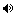listen again online or download the podcast. It is a useful general introduction to the Fibonacci Numbers and the Golden Section.
• Fibonacci Numbers and Nature
Fibonacci and the original problem about rabbits where the series first appears, the family trees of cows and bees, the golden ratio and the Fibonacci series, the Fibonacci Spiral and sea shell shapes, branching plants, flower petal and seeds, leaves and petal arrangements, on pineapples and in apples, pine cones and leaf arrangements. All involve the Fibonacci numbers - and here's how and why.
• The Golden section in Nature
Continuing the theme of the first page but with specific reference to why the golden section appears in nature. Now with a Geometer's Sketchpad dynamic demonstration.

0, 1, 1, 2, 3, 5, 8, 13, 21, 34, 55, 89, 144, 233, 377, 610, 987 ..More..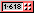### The Puzzling World of Fibonacci Numbers

A pair of pages with plenty of playful problems to perplex the professional and the part-time puzzler!
• The Easier Fibonacci Puzzles page
has the Fibonacci numbers in brick wall patterns, Fibonacci bee lines, seating people in a row and the Fibonacci numbers again, giving change and a game with match sticks and even with electrical resistance and lots more puzzles all involve the Fibonacci numbers!
• The Harder Fibonacci Puzzles page
still has problems where the Fibonacci numbers are the answers - well, all but ONE, but WHICH one? If you know the Fibonacci Jigsaw puzzle where rearranging the 4 wedge-shaped pieces makes an additional square appear, did you know the same puzzle can be rearranged to make a different shape where a square now disappears?
For these puzzles, I do not know of any simple explanations of why the Fibonacci numbers occur - and that's the real puzzle - can you supply a simple reason why??

### The Intriguing Mathematical World of Fibonacci and Phi

The golden section numbers are also written using the Greek letters Phi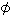and phi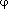.
• The Mathematical Magic of the Fibonacci numbers
looks at the patterns in the Fibonacci numbers themselves: the Fibonacci numbers in Pascal's Triangle; using the Fibonacci series to generate all right-angled triangles with integers sides based on Pythagoras Theorem. If you want to look like a number wizard to your friends then try out the simple Fibonacci numbers trick!
The following pages give you lots of opportunities to find your own patterns in the Fibonacci numbers. We start with a complete list of...

### The Golden Section

The golden section number is closely connected with the Fibonacci series and has a value of (√5 + 1)/2 or:

1·61803 39887 49894 84820 45868 34365 63811 77203 09179 80576 ..More..which we call Phi (note the capital P), the Greek letter Φ, on these pages. The other number also called the golden section is Phi-1 or 0·61803... with exactly the same decimal fraction part as Phi. This value we call phi (with a small p), the Greek letter φ here. Phi and phi have some interesting and unique properties such as 1/phi is the same as 1+phi=Phi.
The third of Simon Singh's Five Numbers programmes broadcast on 13 March 2002 on BBC Radio 4 was all about the Golden Ratio. It is an excellent introduction to the golden section. I spoke on it about the occurrence in nature of the golden section and also the Change Puzzle.Hear the whole programme (14 minutes) using the free RealOne Player.

• The Golden section - the Number
The golden section is also called the golden ratio, the golden mean and Phi.

Two more pages look at its applications in Geometry: first in flat (or two dimensional) geometry and then in the solid geometry of three dimensions.
The next pages are about the numbers Phi = 1·61803.. and phi = 0·61803... and their properties.
• Phi's Fascinating Figures - the Golden Section number
All the powers of Phi are just whole multiples of itself plus another whole number. Did you guess that these multiples and the whole numbers are, of course, the Fibonacci numbers again? Each power of Phi is the sum of the previous two - just like the Fibonacci numbers too.
• Introduction to Continued Fractions
is an optional page that expands on the idea of a continued fraction (CF) introduced in the Phi's Fascinating Figures page.
• There is also a Continued Fractions Converter (a web page - needs no downloads or special plug-is) to change decimal values, fractions and square-roots into and from CFs.
• This page links to another auxiliary page on Simple Exact Trig values such as cos(60°)=1/2 and finds all simple angles with an exact trig expression, many of which involve Phi and phi.
• Phigits and Base Phi Representations
We have seen that using a base of the Fibonacci Numbers we can represent all integers in a binary-like way. Here we show there is an interesting way of representing all integers in a binary-like fashion but using only powers of Phi instead of powers of 2 (binary) or 10 (decimal).

### The Golden String

The golden string is also called the Infinite Fibonacci Word or the Fibonacci Rabbit sequence. There is another way to look at Fibonacci's Rabbits problem that gives an infinitely long sequence of 1s and 0s called the Golden String:-
1 0 1 1 0 1 0 1 1 0 1 1 0 1 0 1 1 0 1 ...

This string is a closely related to the golden section and the Fibonacci numbers.
• Fibonacci Rabbit Sequence
See show how the golden string arises directly from the Rabbit problem and also is used by computers when they compute the Fibonacci numbers. You can hear the Golden sequence as a sound track too.
The Fibonacci Rabbit sequence is an example of a fractal - a mathematical object that contains the whole of itself within itself infinitely many times over.

### Fibonacci - the Man and His Times

• Who was Fibonacci?
Here is a brief biography of Fibonacci and his historical achievements in mathematics, and how he helped Europe replace the Roman numeral system with the "algorithms" that we use today.
Also there is a guide to some memorials to Fibonacci to see in Pisa, Italy.

### More Applications of Fibonacci Numbers and Phi

• The Fibonacci numbers in a formula for Pi ()
There are several ways to compute pi (3·14159 26535 ..) accurately. One that has been used a lot is based on a nice formula for calculating which angle has a given tangent, discovered by James Gregory. His formula together with the Fibonacci numbers can be used to compute pi. This page introduces you to all these concepts from scratch.
• Fibonacci Forgeries
Sometimes we find series that for quite a few terms look exactly like the Fibonacci numbers, but, when we look a bit more closely, they aren't - they are Fibonacci Forgeries.
Since we would not be telling the truth if we said they were the Fibonacci numbers, perhaps we should call them Fibonacci Fibs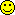!!
• The Lucas Numbers
Here is a series that is very similar to the Fibonacci series, the Lucas series, but it starts with 2 and 1 instead of Fibonacci's 0 and 1. It sometimes pops up in the pages above so here we investigate it some more and discover its properties.
It ends with a number trick which you can use "to impress your friends with your amazing calculating abilities" as the adverts say. It uses facts about the golden section and its relationship with the Fibonacci and Lucas numbers.

2, 1, 3, 4, 7, 11, 18, 29, 47, 76, 123, 199, 322, 521, 843 ....• The Fibonomials
The basic relationship defining the Fibonacci numbers is F(n) = F(n – 1) + F(n – 2) where we use some combination of the previous numbers (here, the previous two) to find the next. Is there such a relationship between the squares of the Fibonacci numbers F(n)2? or the cubes F(n)3? or other powers? Yes there is and it involves a triangular table of numbers with similar properties to Pascal's Triangle and the binomial numbers: the Fibonomials.
• General Fibonacci Series
The Lucas numbers change the two starting values of the Fibonacci series from 0 and 1 to 2 and 1. What if we changed these to any two values? These General Fibonacci series are called the G series but the Fibonacci series and Phi again play a prominent role in their mathematical properties. Also we look at two special arrays (tables) of numbers, the Wythoff array and the Stolarsky array and show how a these two collections of general Fibonacci series contain each whole number exactly once. The secret behind such clever arrays is ... the golden section number Phi!

### Fibonacci and Phi in the Arts

• Fibonacci Numbers and The Golden Section In Art, Architecture and Music
The golden section has been used in many designs, from the ancient Parthenon in Athens (400BC) to Stradivari's violins. It was known to artists such as Leonardo da Vinci and musicians and composers, notably Bartók and Debussy. This is a different kind of page to those above, being concerned with speculations about where Fibonacci numbers and the golden section both do and do not occur in art, architecture and music. All the other pages are factual and verifiable - the material here is a often a matter of opinion. What do you think?

### Awards for this WWW site

Each icon is a link to lists of other Award winning sites that opens in a new window. Check them out!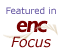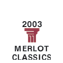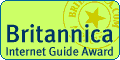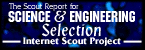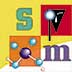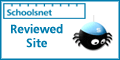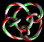Other citations

In association with amazon.com and amazon.co.uk - on-line sources for ordering books recommended at this site.
As an Amazon Associate I earn from qualifying purchases.
For a complete list
and ordering (from USA):
If you are in the UK, try:
 Search: Books Popular Music Classical Music Video Toys Electronics Keywords: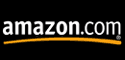Search: All Products Books DVD & Video Popular Music Classical Music Software PC and Video Games Keywords:This site went live in March 1996 and is therefore the oldest maths site on the web!
Hosted by the Department of Mathematics, Surrey University, Guildford, UK, where the author was a Lecturer in the Mathematics and Computing departments 1979-1998.

Blast From the Past: An archived snapshot of this site as it was in June 1998 and at various times from 1999 to 2005 from www. archive.org!
© 1996-2018 Dr Ron Knott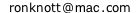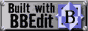Created (March) 1996,